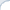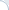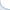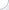Many people complain that there is no simple statistical interpretation for the TF-IDF ranking formula (the formula that is commonly used in information retrieval). However, it can be easily shown that the TF-IDF ranking is based on the distance between two probability distributions, which is expressed as the cross-entropy One is the global distribution of query words in the collection and another is a distribution of query words in documents. The TF-IDF ranking is a measure of perplexity between these two distributions. If the distribution of query words in a document is unusual given the distribution of words in the collection, this is unlikely to happen by chance. In other words, if the global distribution of words is perplexed by the distribution of query words in a document, such document can be relevant. Furthermore, a larger perplexity score implies higher potential relevance of the document.

Let's do a bit of math. The cross-entropy between distributions $p_i$ and $q_i$ is as follows:
$$- \sum_i p_i \log q_i = \sum_i p_i \log \frac{1}{q_i}$$
If you substitute pi with a relative term frequency in a document (normalized by a document length) and $\frac{1}{q_i}$ with the inverted probability of encountering a document with a query term number i, you immediately obtain a TF-IDF formula. From a course in language statistics, we know that estimating probabilities using frequency can be inaccurate. Hence, smoothing is typically used. Many TF-IDF formuals, such as BM25 differ only in the way you smooth your language models. Yet, many (but not all) ranking formulas are essentially cross-entropy estimates. Croft and Lafferty discuss this topic in detail.

Another elephant in the room is that proximity of query terms in a document can also be computed using the cross-entropy. Instead of individual words, however, we need to compute probability distributions of gapped q-grams. A gapped q-gram is a pair of word separated by zero or more other words. Intuitively, we are interested only in pairs where words are sufficiently close to each other. Two major approaches exist. We can either completely ignore pairs where the distance between words is above a threshold, e.g., 10. Alternatively, we can use a kernel function that multiplicatively modifies the income of a gapped q-gram to the overall document score. The value of the kernel function decreases as the distance between words increases (and typically approaches zero when the distance surpasses 10-20). Why 10-20? I think this is related to sentence length: a pair of word is relevant when it occurs in a sentence (or in close sentences). Relevance of non-close pairs is captured well by bag-of-word models.

Metzler and Croft demonstrated that such models can be effective. Still, there is a controversy as to whether such methods work. According to our experience, gapped n-gram models can give you a 20-30% improvement over BM25. In addition, the simple threshold-based model for gapped n-grams works apparently as well as the kernel-based approaches. See, our report for details.

Then one could use cross-extropy to construct a formula similar to BM25 and compare it to the classic one.
For cross-extropy it wll be (1 - p_i) instead of p_i and (1 - q_i) instead of q_i.

Why should this be 1-p? Cross entropy is defined for distributions, not for their complements.

Right, but cross extropy is different to cross entropy in that it's defined for complements. I mean perhaps no one has tested a function similar to BM25 constructed on cross extropy.

Ahhh, I see. cross-EXTROPY. No, I haven't seen anyone using it.